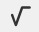PSABPUPG - Cool-Down---Acc7.3 Lesson 6 Distinguishing between Two Types of Situations (7.EE.B.3)
Part A)

Five students came for after-school tutoring. Lin’s teacher assigned each of them the same number of problems to complete. Then he assigned each student 2 more problems. 30 problems were assigned in all.

Write an equation for the story. Write the equation using the "WIRIS editor" buttonPart B)

Find the number of problems originally assigned by Lin's teacher.

If you get stuck, try drawing a diagram to represent the story.

Type your answer below as a number (example: 5, 3.1, 4 1/2, or 3/2):
Part A)

Five students came for after-school tutoring. Priya’s teacher assigned each of them the same number of problems to complete. Then she assigned 2 more problems to one of the students. 27 problems were assigned in all.

Write an equation for the story. Write the equation using the "WIRIS editor" button